# Context-adaptive binary arithmetic coding . Part 2

Context-adaptive binary arithmetic coding . Part 2

### CHAPTER 7### Arithmetic coding procedure as a flow diagram

We will now try to present the steps of the arithmetic coding procedure as a flow diagram. Let the alphabet of the message to be transmitted consist of the character set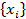(in the examples above, this alphabet consisted of three characters,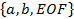, with i = 1 for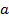, i = 2 for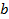, and i = 3 for EOF). Construct an array of values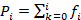, where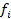is the relative frequency of the i-th character in the message. Let= 0 and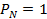, where N is the number of characters in the alphabet. (Again, in the coding example discussed above, we have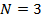and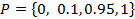.) Using the notation thus introduced, the steps to be performed during arithmetic coding of each character can be presented as a flow diagram shown in Fig. 1.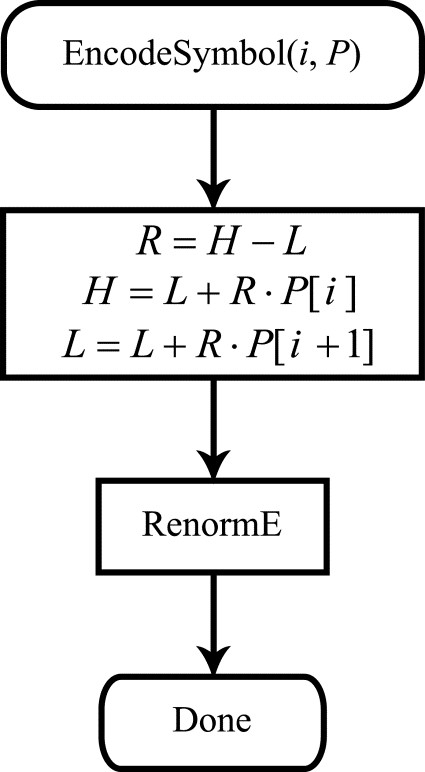Figure 1. Flow diagram of the arithmetic coding procedure

The EncodeSymbol() procedure takes two arguments: i, the number of the character to be coded in the alphabet, and P, the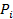array. As can be seen from the flow diagram, the first coding step consists of calculating the current interval length(using the current values of the left and right interval endpoints,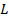andrespectively). The quantityis used to calculate the updated values of the interval endpoints. Thus, in the first coding step for each message character, we split the current segment. The second step consists of a renormalization procedure, shown as a flow diagram in Fig. 2.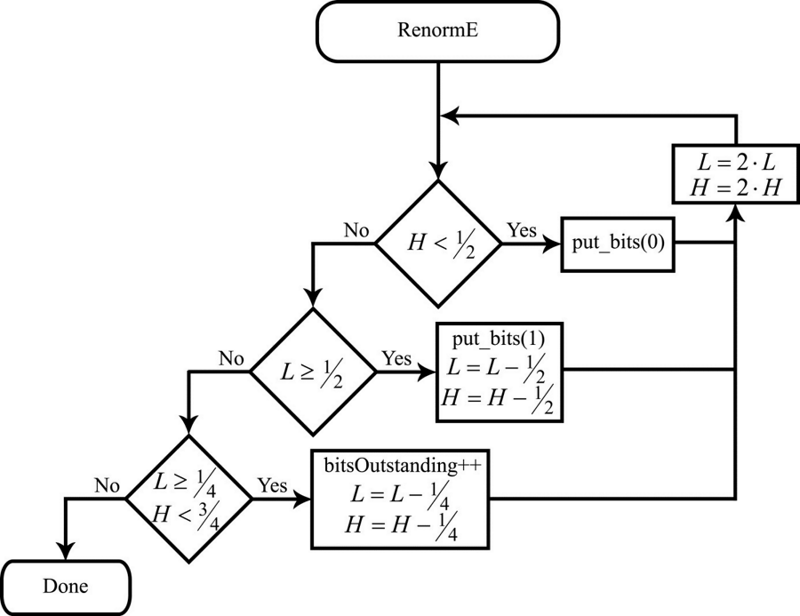Figure 2. Flow diagram of the renormalization procedure

As already noted when discussing renormalization, if the current interval is fully contained inside the range [0, 1), i.e. if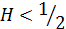, a zero is output to the resulting bitstream followed by a sequence of ones equal in length to the value of the bitsOutstanding variable. All of this is carried out inside the put_bits() procedure, shown as a flow diagram in Fig. 4. The interval endpoint values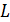and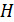are doubled.

If the current interval is fully contained inside the range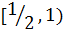, i.e. if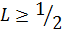, a one is output to the resulting bitstream followed by a sequence of zeros equal in length to the value of the bitsOutstanding variable (put_bits() is again used here). The interval endpoints in this case are modified so as to double the distance fromandto 1. Therefore, the updated values ofandcan be calculated as: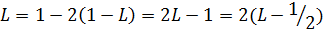,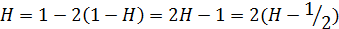,

which is carried out in this branch of the RenormE procedure.

Finally, if the current interval is fully contained inside the range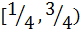, i.e. if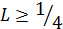, and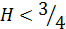, the value of bitsOutstanding is incremented by 1, and the interval endpoints are shifted towards each other so as to double the distance fromandto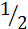: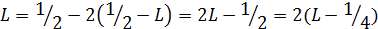,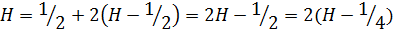,

which is carried out in this branch of the RenormE procedure.

The flow diagram of the put_bits() procedure is shown in Fig. 4. This procedure takes the bit value (0 or 1) as an argument and outputs it to the resulting bitstream that represents the result of the arithmetic coding. The procedure of outputting the bit to the stream is designated as write_bit() in the flow diagram. After completing the output, the value of bitsOutstanding is checked. A sequence of !b values (here, the flow diagram uses the syntax for the Boolean NOT operation from the C language) equal in length to the value of bitsOutstanding is output to the bitstream.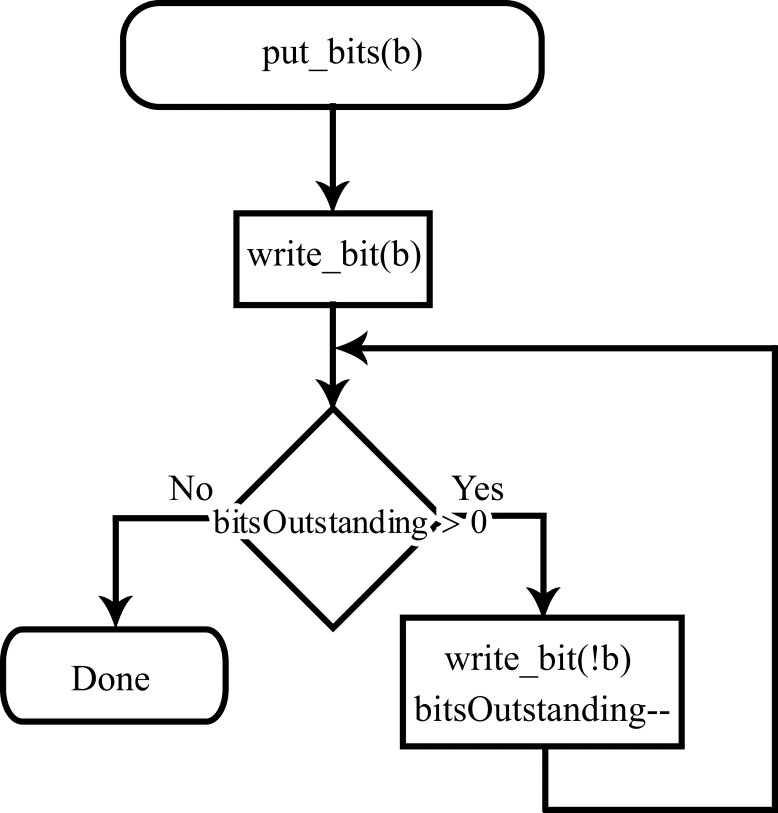Figure 3. Flow diagram of the put_bits() procedure

June 13, 2019

Chapter 1. Video encoding in simple terms

Chapter 2. Inter-frame prediction (Inter) in HEVC

Chapter 3. Spatial (Intra) prediction in HEVC

Chapter 4. Motion compensation in HEVC

Chapter 5. Post-processing in HEVC